# Naive Forecasts¶

In some sense, time series forecasting is easy, if we have low expectations. From a dynamical system point of view, our future is usually not too different from our current state.

## Last Observation¶

Assuming our time series is not changing dramatically, we can take our last observation as our forecast.

Example: Last Observation as Forecast

Assuming we have the simplest dynamical system,

$\frac{\mathrm d y(t)}{\mathrm dt} = f(y, t, \theta),$

where $$y(t)$$ is the time series generator function, $$t$$ is time, $$\theta$$ is some parameters defining the function $$f$$.

For example,

$\frac{\mathrm d y(t)}{\mathrm dt} = t$

is a linear growing time series.

We would imagine, it won't be too crazy if we just take the last observed value as our forecast.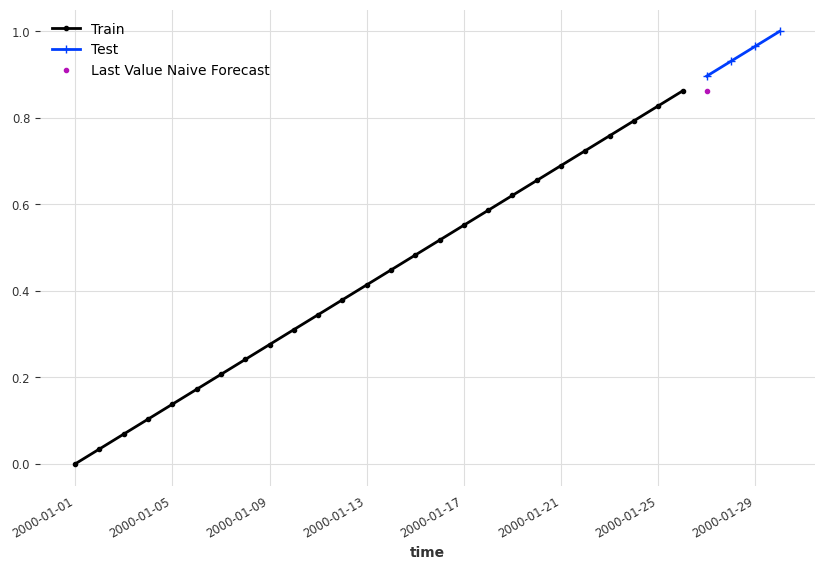import matplotlib.pyplot as plt

from darts.utils.timeseries_generation import linear_timeseries

ts = linear_timeseries(length=30)
ts.plot(marker=".")

ts_train, ts_test = ts.split_before(0.9)
ts_train.plot(marker=".", label="Train")
ts_test.plot(marker="+", label="Test")

ts_last_value_naive_forecast = ts_train.shift(1)[-1]

fig, ax = plt.subplots(figsize=(10, 6.18))
ts_train.plot(marker=".", label="Train", ax=ax)
ts_test.plot(marker="+", label="Test", ax=ax)
ts_last_value_naive_forecast.plot(marker=".", label="Last Value Naive Forecast")


There are also slightly more complicated naive forecasting methods.

## Mean Forecast¶

In some bounded time series, the mean of the past values is also a good naive candidate1.

Example: Naive Mean Forecast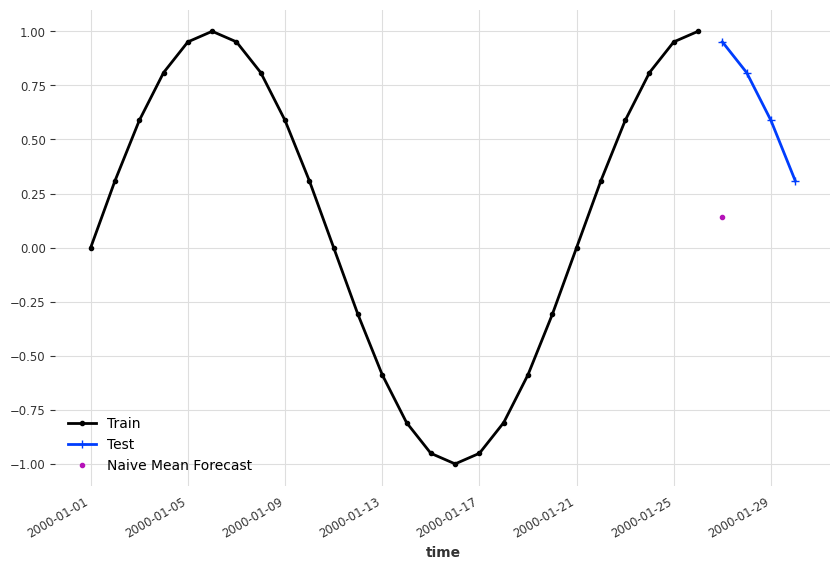import matplotlib.pyplot as plt

from darts.utils.timeseries_generation import sine_timeseries
from darts.models.forecasting.baselines import NaiveMean

ts_sin = sine_timeseries(length=30, value_frequency=0.05)

ts_sin.plot(marker=".")

ts_sin_train, ts_sin_test = ts_sin.split_before(0.9)

ts_sin_train.plot(marker=".", label="Train")
ts_sin_test.plot(marker="+", label="Test")

naive_mean_model = NaiveMean()
naive_mean_model.fit(ts_sin_train)
ts_mean_naive_forecast = naive_mean_model.predict(1)

fig, ax = plt.subplots(figsize=(10, 6.18))
ts_sin_train.plot(marker=".", label="Train", ax=ax)
ts_sin_test.plot(marker="+", label="Test", ax=ax)
ts_mean_naive_forecast.plot(marker=".", label="Naive Mean Forecast")


## Simple Exponential Smoothing¶

Simple Exponential Smoothing (SES) is a naive smoothing method to account for the historical values of a time series when forecasting. The expanded form of SES is1

$y(t) = \alpha ( y(t-1) + (1-\alpha) y(t-2) + (1-\alpha)^2 y(t-3) + \cdots )$

Truncated SES is Biased

Naively speaking, if history is constant, we have to forecast the same constant. For example, if we have $$y(t) = y(t_0)$$, the smoothing

$y(t) = \alpha (1 + (1-\alpha) + \cdots) y(t_0)$

should equal to $$y(t_0)$$, i.e.,

$\alpha (1 + (1-\alpha) + \cdots) = 1.$

The series indeed sums up to $$1/\alpha$$ when $$n\to\infty$$ since

$\sum_{n=0}^\infty (1-\alpha) = \frac{1}{\alpha}.$

However, if we truncate the series to finite values, we will have

$\sum_{n=0}^N (1-\alpha) \leq \frac{1}{\alpha}.$

Then our naive forecast for constant series is

$y(t) = \alpha \sum_{n=0}^N (1-\alpha) y(t_0) \leq y(t_0),$

when $$y(t_0)$$ is positive.

As an intuition, we plot out the sum of the coefficients for different orders and $$\alpha$$s.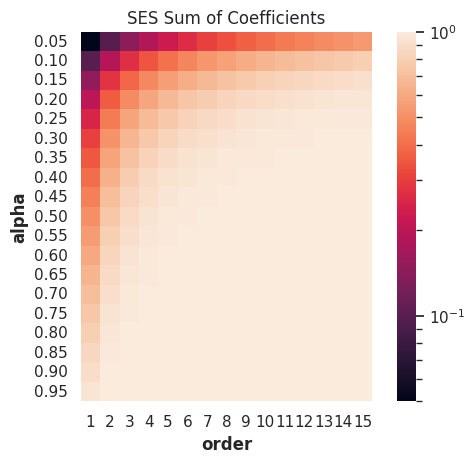from itertools import product
import pandas as pd
import seaborn as sns; sns.set()
from matplotlib.colors import LogNorm

def ses_coefficients(alpha, order):
return (
np.power(
np.ones(int(order)) * (1-alpha), np.arange((order))
) * alpha
)

alphas = np.linspace(0.05, 0.95, 19)
orders = list(range(1, 16))

# Create dataframes for visualizations
df_ses_coefficients = pd.DataFrame(
[[alpha, order] for alpha, order in product(alphas, orders)],
columns=["alpha", "order"]
)
df_ses_coefficients["ses_coefficients_sum"] = df_ses_coefficients.apply(
lambda x: ses_coefficients(x["alpha"], x["order"]).sum(), axis=1
)

# Visualization
g = sns.heatmap(
data=df_ses_coefficients.pivot(
"alpha", "order", "ses_coefficients_sum"
),
square=True, norm=LogNorm(),
fmt="0.2g",
yticklabels=[f"{i:0.2f}" for i in alphas],
)

g.set_title("SES Sum of Coefficients");


Holt-Winters' Exponential Smoothing

In applications, the Holt-Winters' exponential smoothing is more practical123.

We created some demo time series and apply the Holt-Winters' exponential smoothing. To build see where exponential smoothing works, we forecast at different dates.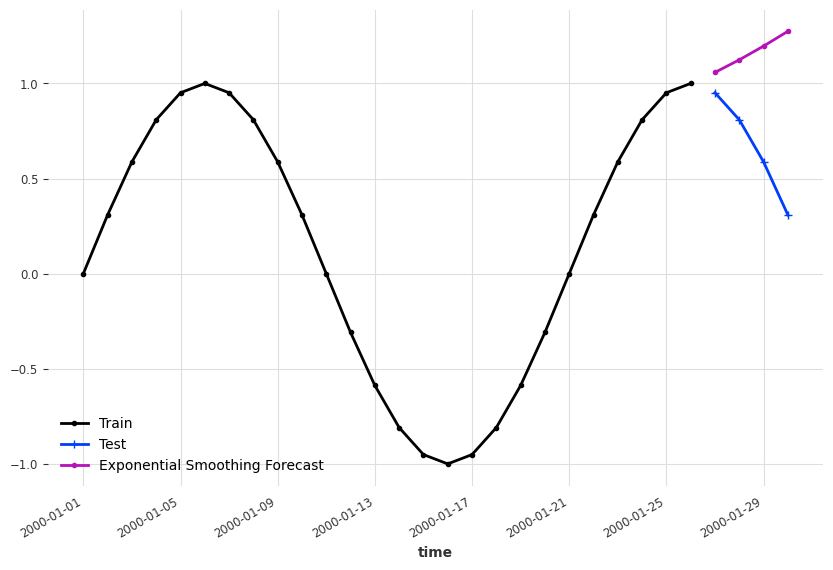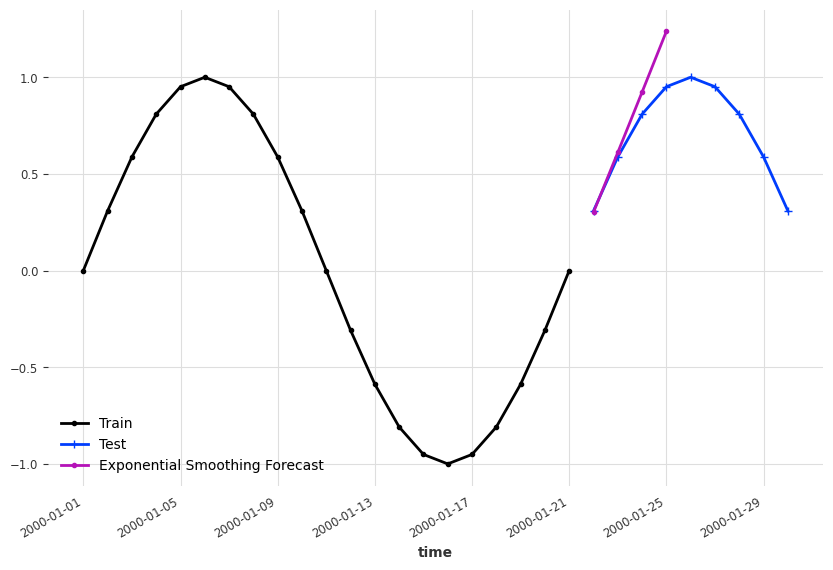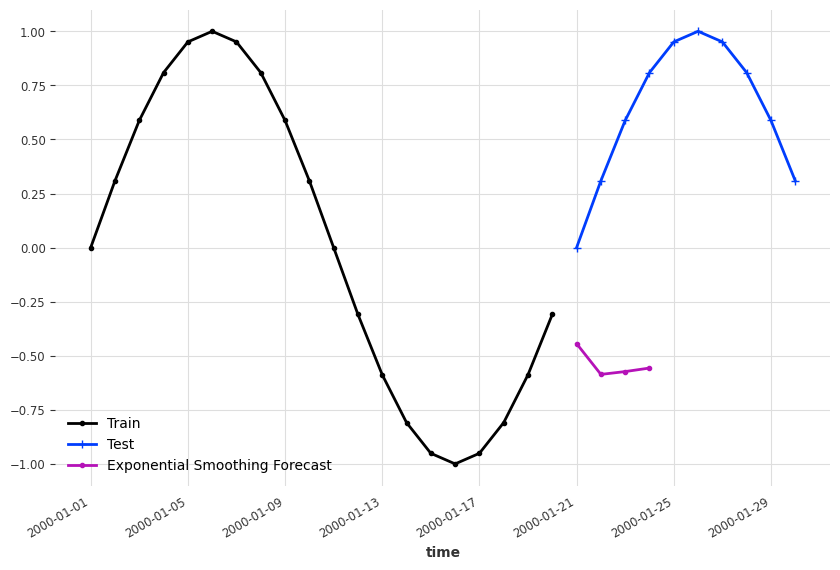import matplotlib.pyplot as plt

from darts.utils.timeseries_generation import sine_timeseries
from darts.models.forecasting.baselines import NaiveMean

ts_sin = sine_timeseries(length=30, value_frequency=0.05)

ts_sin.plot(marker=".")

ts_sin_train, ts_sin_test = ts_sin.split_before(0.7)

es_model = ExponentialSmoothing()

es_model.fit(ts_sin_train)
es_model_sin_forecast = es_model.predict(4)

fig, ax = plt.subplots(figsize=(10, 6.18))
ts_sin_train.plot(marker=".", label="Train", ax=ax)
ts_sin_test.plot(marker="+", label="Test", ax=ax)
es_model_sin_forecast.plot(marker=".", label="Exponential Smoothing Forecast")


## Other¶

Other naive forecasts, such as naive drift, are introduced in Hyndman, et al., (2021)1.

1. Hyndman, R.J., & Athanasopoulos, G. (2021) Forecasting: principles and practice, 3rd edition, OTexts: Melbourne, Australia. OTexts.com/fpp3. Accessed on 2023-02-13.

2. 6.4.3.5. Triple Exponential Smoothing. In: NIST Engineering Statistics Handbook [Internet]. [cited 16 Feb 2023]. Available: https://www.itl.nist.gov/div898/handbook/pmc/section4/pmc435.htm

3. Example: Holt-Winters Exponential Smoothing — NumPyro  documentation. In: NumPyro [Internet]. [cited 16 Feb 2023]. Available: https://num.pyro.ai/en/stable/examples/holt_winters.html

Contributors: LM